## Understanding Area of Circle with Examples

Practice Area Questions

#### What is Area of Circle?

Area of a cirlce   =   π   ×   r2

The space occupied by a circle in 2-dimensional space is the area of a circle. For derivation of the formula see the section below.

#### Derivation of the formula for area of a circle.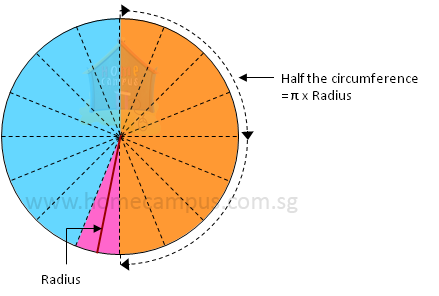Trace a circular shape on paper and cut it along the edge. Fold it in half, then fold it 3 more times so you have 16 folding lines meeting at the centre like below.

Cut the circle along the folding lines to get 16 slices (sectors) and arrange them as below.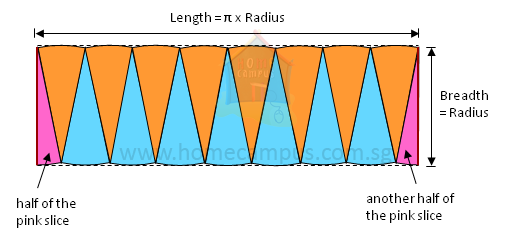The circle is roughly arranged into a rectangle so their areas are now the same.

Area of the circle = Area of the rectangle

## Area of a Circle - Solved Examples

#### 1. What is the area of a circular field of radius 49 m?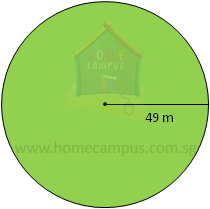Area  =
 22 17
×  497 × 49
=  7546 m2

#### 2. The diameter of a circular frisbee is 24 cm. Find its area. Give your answer in terms of π.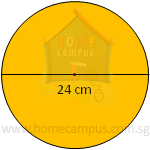Diameter  =  24 cm
=  12 cm
Area  =  π × 12 × 12
=  144 π cm2

#### 3. The diameter of a semicircle is 5 cm. What is its area?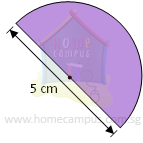Radius  =  5 cm ÷ 2
=  2.5 cm

Area of a semicircle  =  Half of the area of the circle
=
 1 2
× 3.14 × 2.5 × 2.5
=  9.81 cm2

#### 4. Find the area of a quadrant whose radius is 7 m.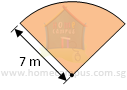Area of a quadrant  =  A quarter of the area of the circle
=
 1 24
×
 1122 17
× 71 × 7
=  38.5 m2

#### 5. Find the area of the fan below made of 4 identical quadrants and a circle.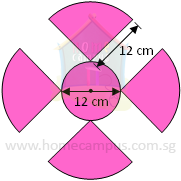Area of the middle circle  =  3.14 × 6 × 6
=  113.04 cm2